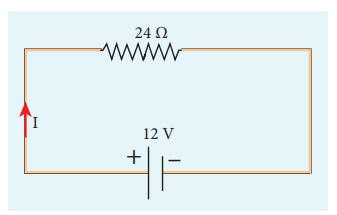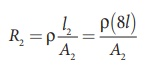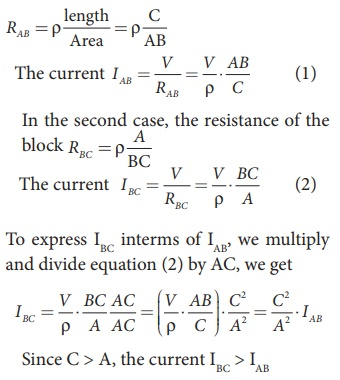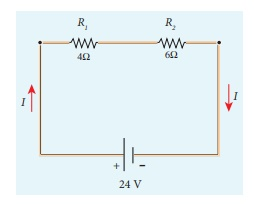Home | | Physics 12th Std | Ohm‚Äôs Law: Solved Example Problems

# Ohm‚Äôs Law: Solved Example Problems

Physics : Current Electricity: Ohm‚Äôs Law: Solved Example Problems

## OHM‚ÄôS LAW: Solved Example Problems

EXAMPLE 2.5

A potential difference across 24 Œ© resistor is 12 V. What is the current through the resistor?

SolutionV = 12 V and R = 24 Œ©

Current, I = ?

From Ohm‚Äôs law, I = V/R = 12/24 = 0 .5 A

## Resistivity: Solved Example Problems

EXAMPLE 2.6

The resistance of a wire is 20 Œ©. What will be new resistance, if it is stretched uniformly 8 times its original length?

Solution

R1 = 20 Œ©, R2= ?

Let the original length (l1) be l.

The new length, l2 = 8l1 (i.,e) l2 =8l

The original resistance, R = œÅ [ l1 / A1]

The new resistance

R2 =Though the wire is stretched, its volume is unchanged.

Initial volume = Final volume

A1l1= A2l2 , A1l = A28l

A1 / A= 8l / l = 8

By dividing equation R2 by equation R1, we getSubstituting the value of A1/A2, we get

R2 / R = 8 √ó8 = 64 2

R2 = 64 √ó 20=1280 Œ©

Hence, stretching the length of the wire has increased its resistance.

EXAMPLE 2.7

Consider a rectangular block of metal of height A, width B and length C as shown in the figure.If a potential difference of V is applied between the two faces A and B of the block (figure (a)), the current IAB is observed. Find the current that flows if the same potential difference V is applied between the two faces B and C of the block (figure (b)). Give your answers in terms of IAB.

Solution

In the first case, the resistance of the block## Resistors in series and parallel: Solved Example Problems

EXAMPLE 2.8

Calculate the equivalent resistance for the circuit which is connected to 24 V battery and also find the potential difference across 4 Œ© and 6 Œ© resistors in the circuit.Solution

Since the resistors are connected in series, the effective resistance in the circuit

The Current I in the circuit= V/ Req = 24/10 = 2 .4 A

V1 = IR1 = 2 . 4 A√ó 4 Œ© = 9.6V

V2 = IR1 = 2 . 4 A√ó 6 Œ© =14 .4V

EXAMPLE 2.9

Calculate the equivalent resistance in the following circuit and also find the current I, I1 and I2 in the given circuit.Solution

Since the resistances are connected in parallel, therefore, the equivalent resistance in the circuit isThe resistors are connected in parallel, the potential (voltage) across each resistor is the same.The current I is the total of the currents in the two branches. Then,

I = I1 + I2= 6 A + 4 A = 10 A

EXAMPLE 2.10

When two resistances connected in series and parallel their equivalent resistances are 15 Œ© and 56/15 Œ© respectively. Find the individual resistances.

Solution

Rs = R1 + R2 = 15 Œ© (1)The above equation can be solved using factorisation.

R12-8 R1-7 R1+ 56 = 0

R1 (R1‚Äì 8) ‚Äì 7 (R1‚Äì 8) = 0

(R1‚Äì 8) (R1‚Äì 7) = 0

using in equation (1)

8 + R2 = 15

R2 = 15 ‚Äì 8 = 7 Œ© ,

Substituting in equation (1)

7 + R2 = 15

R2 = 8 Œ© , i.e , (when R1 = 8 Œ© ; R2 = 7 Œ© )

EXAMPLE 2.11

Calculate the equivalent resistance between A and B in the given circuit.SolutionEXAMPLE 2.12

Five resistors are connected in the configuration as shown in the figure. Calculate the equivalent resistance between the points a and b.Solution

Case (a)

To find the equivalent resistance between the points a and b, we assume that current is entering the junction a. Since all the resistances in the outside loop are the same (1Œ©), the current in the branches ac and ad must be equal. So the electric potential at the point c and d is the same hence no current flows into 5 Œ© resistance. It implies that the 5 Œ© has no role in determining the equivalent resistance and it can be removed. So the circuit is simplified as shown in the figure.The equivalent resistance of the circuit between a and b is Req = 1Œ©

## Temperature dependence of resistivity: Solved Example Problems

EXAMPLE 2.13

If the resistance of coil is 3 Œ© at 20 0C and Œ± = 0.004/0C then determine its resistance at 100 0C.

Solution

R0= 3 Œ©,‚ÄÉ T = 100¬∫C,‚ÄÉ T0 = 20¬∫C

Œ± = 0.004/¬∫C,‚ÄÉ RT = ?

RT= R0(1 + Œ±(T-T0))

R100 = 3(1 + 0.004 √ó 80)

R100 = 3(1 + 0.32)

R100 = 3(1.32)

EXAMPLE 2.14

Resistance of a material at 10¬∫C and 40¬∫C are 45 Œ© and 85 Œ© respectively. Find its temperature co-efficient of resistance.

Solution

T0 = 10¬∫C, T = 40¬∫C, R0= 45 Œ© , R = 85 Œ©

Œ± = 1/R . ŒîR /ŒîTŒ± = 0.0296 per ¬∫C

Study Material, Lecturing Notes, Assignment, Reference, Wiki description explanation, brief detail
12th Physics : Current Electricity : Ohm‚Äôs Law: Solved Example Problems |